0
1095

# TOP-30 Syllogisms for IBPS Clerk

Download Syllogisms Questions PDF based on previously asked questions in IBPS Clerk and other Banking Exams. Practice Syllogisms Questions for IBPS Clerk Exam.

Take Free IBPS Clerk Mock Test

Go to Free Banking Study Material (15,000 Solved Questions)Instructions

In each question below are statements followed by two conclusions numbered I and II You have to take the two given statements to be true even if they seem to be at variance from commonly known facts and then decide which of the given conclusions logically follows from the given statements disregarding commonly known facts.
(a)If only Conclusion I follows

(b)If only Conclusion II follows

(c)If either Conclusion I or II follows

(d)If neither Conclusion I nor II follows

(e)If both Conclusions I and II follow

Question 1: Statements: All roses are flowers. No flower is a plant. No plant is a fruit.
Conclusion:I)No fruit is a flower
II)All fruits can be flowers

a) Only Conclusion I follows

b) Only Conclusion II follows

c) Either Conclusion I or Conclusion II follows

d) Neither Conclusion I nor Conclusion II follows.

e) Both Conclusion I and Conclusion II follows.

Instructions

In each of the questions below are given three statements followed by three conclusions numbered I, II-III. You have to take the given statements to be true even if they seem to be at variance from commonly known facts Read all the conclusions and then decide which of the given conclusions logically follows from three given statements disregarding commonly known facts. Then decide which of the answer 1),2),3),4) and 5) is the correct answer and indicate it on the answer sheet.

Question 2: Statements:All sweets are fruits
No fruits are pencil
Some pencils are glasses
Conclusions: I.Some glasses are sweets
II. Some pencils are sweets
III.No glass is sweet.

a) Only I follows

b) Only II follows

c) Only III follows

d) Only either I or III follows

e) None of these

Question 3: Statements: All roofs are cameras
Some cameras are photographs
Some photographs are stores
Conclusions:I. Some stores are cameras
II. Some stores are roofs
III.Some cameras are roofs.

a) Only I follows

b) Only II follows

c) Only III follows

d) Only either II and III follow

e) None of these

Instructions

In each of the questions below are given four statements followed by three conclusions numbered I, II and III. You have to take the given statements to be true even if they seem to be at variance from commonly known facts. Read all the conclusions and then decide which of the given conclusions logically follows from three given statements disregarding commonly known facts.

Question 4: Statements:Some nails are plates.
Some plates are disks.
All disks are mirrors.
All mirrors are tires.
Conclusions:I. Some tires are plates.
II.Some tires are nails.
III. Some mirrors are plates.

a) Only I and II follows

b) Only I and III follows

c) Only II and III follows

d) All I, II and III follow

e) None of these

Question 5: Statements: All flowers are houses.
All houses are tigers.
All tigers are goats.
Some goats are bullocks.
Conclusions:I. Some goats are flowers.
II. Some tigers are flowers.
III.Some bullocks are tigers.

a) Only I and II follow

b) Only II and III follow

c) Only I and III follow

d) All I, II and III follow

e) None of these

Instructions

In the questions below, there are some statements followed by 2 conclusions. You have to assume the statements to be true even if they are at variance from commonly known facts. Then you have to decide which of the answers is the correct answer.

Question 6: Statements: Some cats are tortoises.
Some tortoises are dogs.
All dogs are snakes.
Conclusions: P) Some snakes are tortoises.
Q) Some dogs are cats.
R) Some snakes are dogs.

a) Only P and R follow

b) Only P and either Q or R follows

c) Only Q and either P or R follows

d) All P, Q and R follow

e) None of these

Question 7: Statements: All snakes are rabbits.
Some rabbits are boars.
Some boars are cockroaches.
Conclusions: P) Some cockroaches are rabbits.
Q) Some boars are snakes.
R) Some snakes are cockroaches.

a) Only P and either Q or R follow

b) Only either Q or R follows

c) Only P and Q follow

d) All follow

e) None of these

Question 8: Statements: All flowers are bulls.
All bulls are bulldogs.
All bulldogs are alligators.
Conclusions: P) Some alligators are flowers.
Q) All flowers are bulldogs.
R) Some bulldogs are flowers.

a) None follows

b) Only P and Q follow

c) Only Q and R follow

d) Only P and R follow

e) All 3 follow

Instructions

Read the following statements and select the suitable conclusions.

Question 9: Statements I: All mangoes are green.
II: Some mangoes are sweet.
Conclusions I: Some green is sweet.
II: No sweet is green.

a) Only conclusion I follows

b) Only conclusion II follows

c) Either conclusion I or II follows

d) Neither conclusion I nor II follows

e) Both the conclusions follow

Question 10: Statements I: Some camels are lions.
II: No birds are camels.
Conclusions I: No birds are lions.
II: Some birds are lions is a possibility

a) Only conclusion I follows

b) Only conclusion II follows

c) Either conclusion I or II follows

d) Neither conclusion I nor II follows

e) Both the conclusions follow

Question 11: Statements I: Some boxes are big.
II: Some boxes are heavy
Conclusions I: No heavy is big.
II: All heavy is big.

a) Only conclusion I follows

b) Only conclusion II follows

c) Either conclusion I or II follows

d) Neither conclusion I nor II follows

e) Both the conclusions follow

Question 12: Statements I: All cars are bikes.
II: All bikes are trains.
Conclusions I: Some cars are trains.
II: All cars are trains.

a) Only conclusion I follows

b) Only conclusion II follows

c) Either conclusion I or II follows

d) Neither conclusion I nor II follows

e) Both the conclusions follow

Instructions

In the following questions, statements are given followed by conclusions. Find the conclusions that necessarily follow from the statements given. The statements can be assumed to be true, irrespective of their truth-value in real life.

Question 13: Statements:1) All lions are fierce.
2) All lions are golden
Conclusions:1)All golden are fierce.
2)All fierce are golden.

a) Only Conclusion 1 follows.

b) Only Conclusion 2 follows.

c) Both Conclusion 1 and Conclusion 2 follow.

d) Either Conclusion 1 or Conclusion 2 follow but both the conclusions do not follow.

e) None of the conclusions follow.

Instructions

In each question below are given some statements followed by four conclusions numbered I, II, III and IV. You have to take the given statements to be true even if they seem at variance from commonly known facts. Read all the conclusions and then decide which of the given conclusions logically follows from the two given statements, disregarding commonly known facts.

Question 14: Statements: I. All gates are flowers.
Some gates are fruits.
Some flowers are clips.
Conclusions: I. Some flowers are fruits.
II. Some clips are fruits.
III. Some clips are gates.
IV. No flower is fruit.

a) Only I follows

b) Only I and IV follow

c) Only II and IV follow

d) Only I and III follow

e) None of these

Question 15: Statements: Some lice are slates
All slates are apples
No apple is car
Conclusions: I. Some cars are slates
II. Some lice are cars
III. Some apples are lice
IV. No car is lice

a) No, any conclusion follows

b) Only II follow

c) Only III follow

d) Only II or IV and III follow

e) None of these

Question 16: Which of the following conclusions can be made from the given statements.
Statements:
All apples are mangoes.
All grapes are apples.
All bananas are mangoes.
Conclusions:
I) All grapes are mangoes
II) All bananas are apples
III) Some apples are bananas

a) Only I

b) Only II

c) Only III

d) Either II or III

e) Both I and II

Question 17: Which of the following conclusions can be made from the given statements.
Statements:
Some cars are buses.
All buses are vehicles.
Some vehicles are boats.
Conclusions:
I) Some buses are boats
II) Some cars are boats
III) Some vehicles are cars

a) Only I

b) Only II

c) Only III

d) Both I and II

e) Both II and III

Instructions

In each of the following questions, there are some statements and some conclusions that follow them. Given that all the statements are true, read all the conclusions and decide which of them logically follow the statements.

Question 18: Statements:
All shirts are jeans.
Some jeans are garments.
All garments are clothes.
Conclusions:
I. Some jeans are clothes.
II. Some shirts are garments.
III. No shirts are clothes.

a) Only I follows

b) Only II and III follows

c) Only I and III follows

d) All I, II and III follow

e) None of these

Instructions

In each question below are given three statements followed by two conclusions numbered I and II. You have to assume everything in the statements to be true even if they seem to be at variance from commonly known facts and then decide which of the given conclusions logically follows from the information given in the statements.

Question 19: Statements:
All drums are banjos.
Some drums are guitars.
No banjo is a flute.
Conclusions:
I. Some guitars are flutes.
II. No guitar is flute.

a) Only conclusion I follows.

b) Only conclusion II follows.

c) There is a possibility that some flutes are banjos.

d) Either conclusion I or conclusion II follows.

Question 20: Statements:
No car is a hotel.
All lodges are hotels.
No lodge is house.Conclusions:
I. Some houses are not lodges.
II. No lodge is a car.

a) There is possibility that all houses are cars.

b) Only conclusion I follows.

c) Only conclusion II follows.

d) Both conclusion I and conclusion II follow.

Instructions

In each group of questions below are two/three statements followed by two conclusions numbered I and II. You have to take the given statements to be true even if they seem to be at variance from commonly known facts and then decide which of the given conclusions logically follows from the two/three statements disregarding commonly known facts.

Question 21: Statements:
All pens are nibs.
All nibs are inks.
No ink is color.
Conclusions:
I. No color is nib.
II. No color is a pen.

a) If only Conclusion I follows

b) If only Conclusion II follows

c) If either Conclusion I or Conclusion II follows

d) If both conclusions I and II follow.

Instructions

In the next five questions, two or three statements are followed by two conclusions. You have to assume the given statements are true even if they are at a variance from commonly known facts and then determine which of the conclusions are implied.

Question 22: Statement: No brochure is a magazine
All magazines are pamphlets.
All pamphlets are booklets.
Conclusions: 1) All pamphlets can never be brochures
2) All brochures are definitely booklets.

a) Only conclusion 1 is implied

b) Only conclusion 2 is implied

c) Either conclusion 1 or 2 is implied.

d) Neither conclusion 1 nor 2 is implied.

e) Both conclusions are implied.

Question 23: Statements: Some bankers are clerks
All clerks are officers
No officer is a manager.
Conclusion: 1) All bankers are managers.
2) No clerk is a manager.

a) Only conclusion 1 is implied

b) Only conclusion 2 is implied

c) Either conclusion 1 or 2 is implied.

d) Neither conclusion 1 nor 2 is implied.

e) Both the conclusions are implied.

Instructions

Each question contains 3 statements. Consider the statements to be true even if they seem to be at variance with the commonly known facts. Select the conclusions that logically follow the given statement.

Question 24: All hens are ducks. Some ducks are crows. All ducks are kiwis.
I. Some kiwis are crows.
II. Some hens are crows.

a) Only conclusion I follows

b) Only conclusion II follows

c) Either conclusion I or conclusion II follows

d) Both conclusion I and conclusion II follow

e) Neither conclusion I nor conclusion II follows

Instructions

In each of the following questions, two statements followed by two conclusions numbered I and II have been given. You have to take the two given statements to be true even if they seem to be at variance from commonly known facts and then decide which of the given conclusions logically follow from the given statements disregarding commonly known facts.

Question 25: Statements :
Some cooks are employees.
Some employees are workers.
Conclusions :
I. All cooks are workers.
II. No cook is a worker.

a) Either Conclusion I or II follows

b) Only Conclusion II follows

c) Only Conclusion I follows

d) Both Conclusions I and II follow

e) Neither Conclusion I nor II follows

Instructions

In each of the questions given below, there are two statements which are followed by 2 conclusions. You have to take the given statements to be true even if they seem to be at variance from the commonly known facts. You have to then decide which of the given conclusions logically follows from the given statements.

Question 26: Statements:
All books are novel
Conclusions:
II. Some papers are novel

a) if only Conclusion I follows.

b) if only Conclusion II follows.

c) if either Conclusion I or II follows.

d) if neither Conclusion I or II follows.

e) if both Conclusions I and II follow.

Instructions

In each of the questions below are given three statements followed by three conclusions numbered I, II and III. You have to take the given statements to be true even if they seem to be at variance from commonly known facts. Read all the conclusions and then decide which of the given conclusions logically follows from the given statements disregarding commonly known facts.

Question 27: Statements :
Some walls are doors.
Some doors are cots.
Some cots are chairs.
Conclusions :
I : Some chairs are doors.
II: Some cots are walls.
III: No chair is a door.

a) Only II follows

b) Only III follows

c) Only either I or III follows

d) Only I follows

e) None of these

Question 28: Statements:
No note is a coin.
Some coins are metals.
All plastics are notes.
Conclusions:
I. No coin is plastic.
II. All plastics being metals is a possibility.

a) If only conclusion I follows

b) If only conclusion II follows

c) If either conclusion I or conclusion II follows

d) If neither conclusion I nor conclusion II follows

e) If both conclusion I and conclusion II follow

Instructions

In each question below are given two/three statements followed by two conclusions numbered I and II. You have to take the given statements to be true even if they seem to be at variance with commonly known facts. Read all the conclusions and then decide which of the given conclusions logically follows from the given statements, disregarding commonly known facts.

a: If either conclusion I or II follows.
b: If both conclusions I and II follow.
c: If only conclusion I follows.
d: If only conclusion II follows.
e: If neither conclusion I nor II follows.

Question 29: Statements :
Some pastries are sandwiches.
Conclusions :
I. At least some cookies are sandwiches.
II. Some sandwiches are biscuits.

a) If either conclusion I or II follows.

b) If both conclusions I and II follow.

c) If only conclusion I follow.

d) If only conclusion II follows.

e) If neither conclusion I nor II follows.

Instructions

In each question below are two or three statements followed by two conclusions numbered I and II. You have,to take the given statement’ to be true even if they seem to be at variance from commonly know facts and then decide which of the given conclusions logically follows from the given statements disregarding commonly known facts.
Give answer a: if the only conclusion I follow.
Give answer b: if only conclusion II follows.
Give, answer c: if either conclusion I or conclusion follows.
Give answer d: if neither conclusion I nor conclusion II follows.
Give answer e: if both conclusion I and conclusion II follow.

Question 30: Statements
Some plants are mountains.
All plants are rivers.
Conclusions
I. All mountains are plants.
II. At least some mountains are rivers.

a) if only conclusion I follow.

b) if only conclusion II follows.

c) if either conclusion I or conclusion follows.

d) if neither conclusion I nor conclusion II follows.

e) if both conclusion I and conclusion II follow.

These two conclusions can be explained with the help of Venn diagrams. From the given Venn-diagram only conclusion II follows.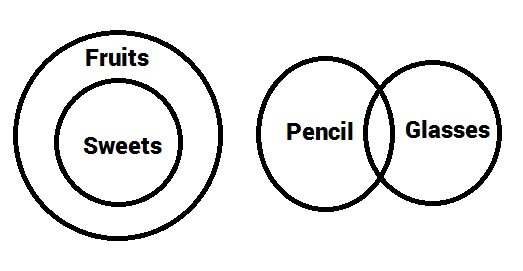We use elimination to find an exception to the generality of the conclusions. Thus we prove they are not implied. The diagram above satisfies all the statements but contradicts the conclusion II. Since we found an exception, the conclusion is not true in “every” case. Thus it is not implied.

Irrespective of the diagram, conclusions I and III are universally complimentary ie. one of the two always has to be implied but both can’t be true at the same time. Hence we use “either” and “or”.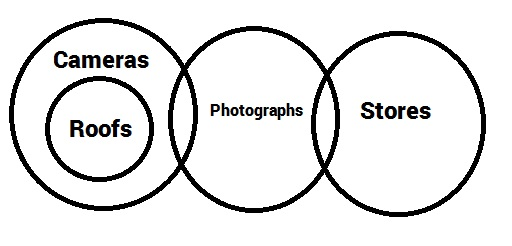We use elimination to find an exception to the generality of the conclusions. Thus we prove they are not implied. The diagram above satisfies all the statements but contradicts the conclusions I and II. Since we found an exception, the conclusions are not true in “every” case. Thus they are not implied.

We can draw many scenarios that satisfy the statements using Venn diagrams and check for the validity of the conclusions. Conclusion III holds in every case. So, it is implied.

The Venn Diagram for the given syllogism is as shown below: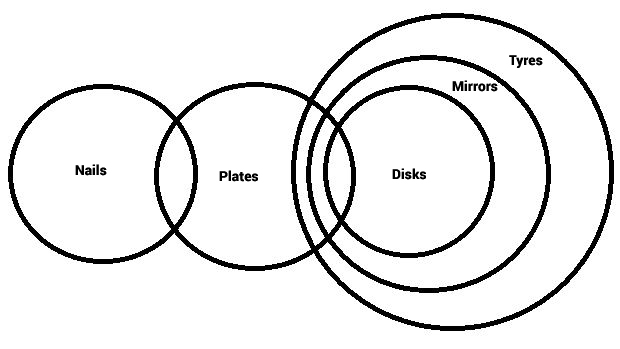Based on the diagram, at least some tires are plates and at least some mirrors are plates. Hence, conclusions I and III are implied. There is no way to confirm that some plates are nails. So, the correct option is option (b).

The Venn Diagram for the given syllogism is as shown below: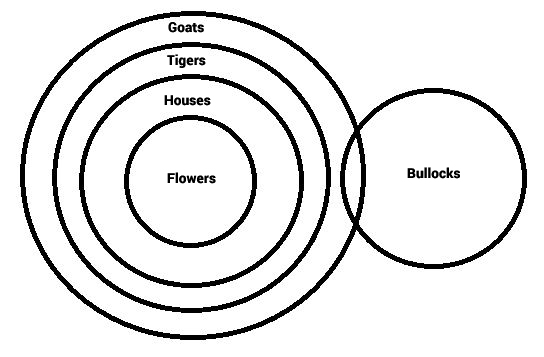From the diagram, at least some goats are flowers and at least some tigers are flowers. Hence conclusions I and II are implied. However, there is no overlap between the circles of bullock and tigers. Hence, it is not implied in every case. So, option (a) is the correct answer.

Based on the information provided, the Venn Diagram is as follows: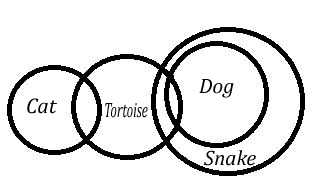Based on the Venn diagram, at least some snakes are tortoises and at least some snakes are dogs. But some dogs are not cats.

Based on the information provided, the Venn Diagram is as follows: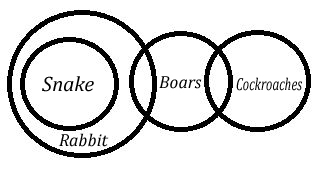Thus, none of the conclusions are implied.

Based on the information provided, the Venn Diagram is as follows: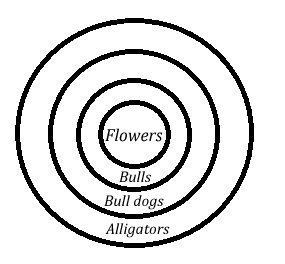Thus, at least some alligators are flowers and at least some bulldogs are flowers and all flowers are bulldogs.

I: All mangoes are green – mango is a subset of green.
II: Some mangoes are sweet – There is some region which is common for both mangoes and sweet.

The Venn diagram for information is as shown:From the Venn diagram, we can see that there is some intersection between green and sweet. Thus, conclusion I is true and II is false.

I: Some camels are lions. – There is some region that is common to both camels and lions.
II: No birds are camels – There is no region common to both birds and camels.

The Venn diagram for the information is given below:From the Venn diagram we can see that some birds are lions is a possibility. Thus, conclusion I am not true and conclusion II is true.

I: Some boxes are big – There is some region common between boxes and big
II: Some boxes are heavy – There is some region common between boxes and heavy

A possible Venn diagram is as shown:From the diagram we can say that some heavy is big hence conclusion I doesn’t follow.
From the diagram we can say that it is not necessary that all heavy is big hence conclusion II doesn’t follow.

From the Venn diagram, we can see that there is some region common between heavy and big. Thus, neither conclusion I nor II is correct.

I: All cars are bikes. – Cars is a subset of bikes
II: All bikes are trains. The bike is a subset of trains. The Venn diagram of the information given is as follows:From the Venn diagram, we can see that all cars are trains. Thus, some cars are trains is definitely true. Hence, conclusion I i true.

Also, from the figure we can see that all cars are trains. Thus, conclusion II is also true.

None of the conclusion can be followed as evident from the following diagram.All lions are fierce means the subset of lions is completely inside the subset of golden. Similarly the subset of lions is completely inside that of fierce. But this is not necessarily the case with the subsets of fierce and golden as shown.

For the conclusions, we use elimination by drawing an exception. Conclusion II, III, IV are contradicted by this diagram below. Only conclusion I is valid. We can draw as many diagrams as needed but I is still valid.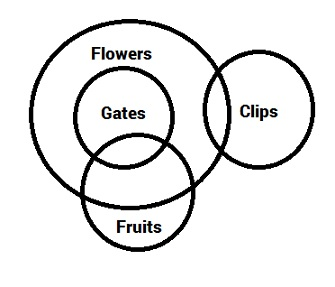Irrespective of the diagram, conclusions II and IV are universally complimentary ie. one of the two has to be true and both can’t be true at the same time. Conclusion III is implied from the diagram. We can draw many other diagrams but III always holds. So, the correct answer is (d)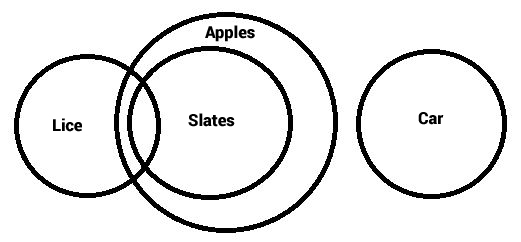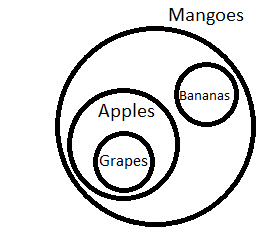From the diagram, we can see that only conclusion I can be made.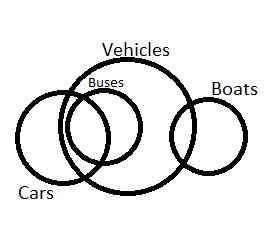From the diagram, we can see that only conclusion III can be made.A and B are two situations where all the given three statements are true.
In situation A, I and III follow while in situation 2 only I follow.
II doesn’t follow in any diagram so it is definitely incorrect, III only follows in A thus we can conclude that it is not necessary that it always follow.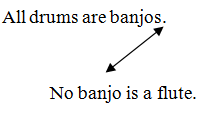A + E => E-type of conclusion

“No drum is a flute.”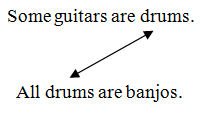I + A => I-type of conclusion
“Some guitars are banjos.”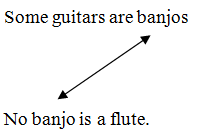I + E O-type of conclusion.

“Some guitars are not flutes.”
Conclusion I and II form complementary pair. So, either conclusion I or conclusion II follows.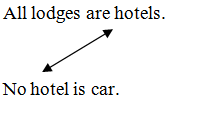A + E => E-type of Conclusion
“No lodge is a car.”
This is Conclusion II.
No lodge is house:
Its Conversion would be
“No house is lodge.”
If no house is lodge, then some houses are also not lodges.
Therefore, Conclusion I also follows.

(i) All pens are nibs Universal Affirmative (A-type).
(ii) Some numbers are digits Particular Affirmative (I-type).
(iii) No ink is colour Universal Negative (E-type).
(iv) Some inks are not colours Particular Negative (O-type).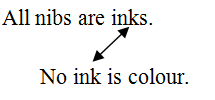A+E => E-type of Conclusion

“No nib is colour.”
Conclusion I is Converse of it.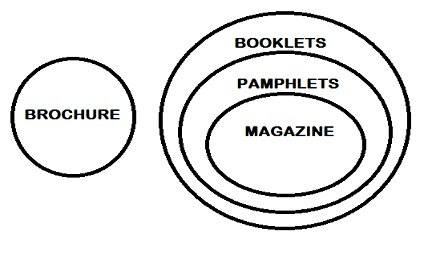All magazines are pamphlets. So, magazine is a subset of pamphlets. Since no magazine is a brochure, we can conclude that all pamphlets can never be brochures.
The diagram shows that “all brochures are definitely booklets” is not implied.

Since no officers is a manager and all clerks are officers, the diagram will be as shown below: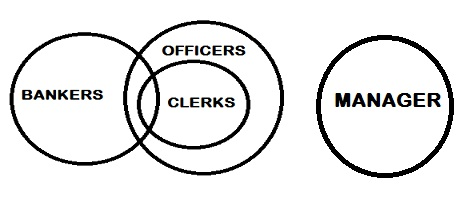The first conclusion is not implied based on the diagram. Hence only the second conclusion ie. “No clerk is a manager” is implied.

Drawing the figure based on the given information:

The venn diagram for above statements is :

Conclusions :

I. All cooks are workers = false
II. No cook is a worker = may or may not be true

Thus, neither Conclusion I nor II follows

=> Ans – (E)

We get the following diagram –

Thus, the conclusion I do not necessarily follow as it is possible that no books are bad. Conclusion II also does not necessarily follow as no paper being novel is a possibility.
Hence, option D is the correct choice.

The Venn diagram for above statements is :

Conclusions :

I. Some chairs are doors = may or may not be true
II. Some cots are walls = false
III. No chair is door = may or may not be true

Thus, only either I or III follows

=> Ans – (C)

All plastics are notes and no note is a coin => no coin is plastic => Conclusion 1 follows.

Some coins are metals => Some notes being metals is a possibility => All plastics being metals is a possibility => Conclusion 2 follows.

=> Both follow

The Venn diagram for above statements is :

Conclusions :

I. At least some cookies are sandwiches = false
II. Some sandwiches are biscuits = false

Thus, neither conclusion I nor II follows.

=> Ans – (E)